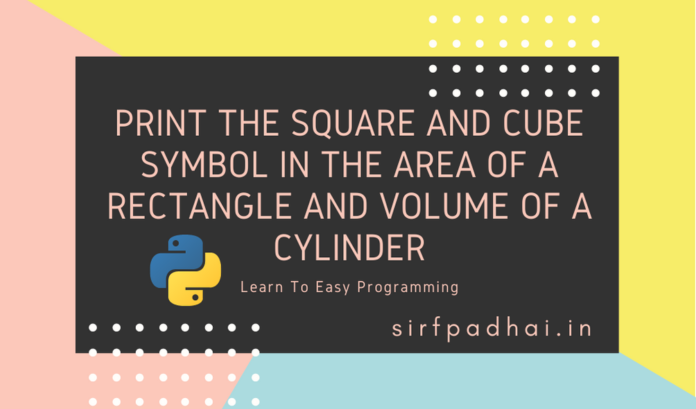# Python: Print the square and cube symbol in the area of a rectangle and volume of a cylinder## Write a python program to print the square and cube symbol in the area of a rectangle and volume of a cylinder.

Python Code:-

``````area = 1256.66
volume = 1254.725
decimals = 2
print("The area of the rectangle is {0:.{1}f}cm\u00b2".format(area, decimals))
decimals = 3
print("The volume of the cylinder is {0:.{1}f}cm\u00b3".format(volume, decimals))``````

Output:-

``````The area of the rectangle is 1256.66cm²
The volume of the cylinder is 1254.725cm³``````

Flowchart:-